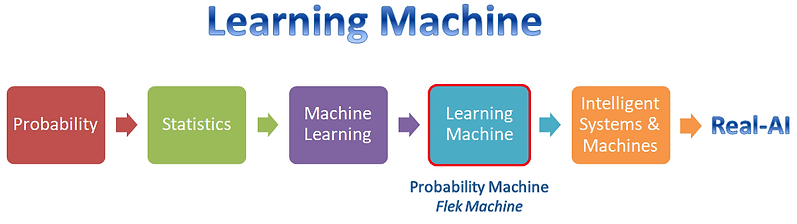top of page

At the core of the Flek Machine runs a Probability Machine - a natural evolution towards Real-AI.

What is a probability machine?

A Probability Machine is a special kind of ML engine that automatically learns a probabilistic models from semi-structured data. It is an invention and a major contribution to the field of AI in general and probability mathematics in particularWhy build a Probability Machine?

Actually, why not build a probability machine and why did it take so long?

Today there are machines that do all sorts of work. Thus, it is a natural step in the road towards real-AI to build a learning machine that takes us a step beyond machine learning. Given the history of probability and its profound influence on statistics and mathematical, we believe that a critical stage in this evolution is to build a Probability Learning Machine.

How does it work?

Contrary to treating models as algorithms or formulas, models built and managed by a probability machine act like database models – i.e. they can be updated, queried and explored for analytical purposes or for making prediction. Also, instead of working with probabilities as distributions, the machine treats them as first class objects – Nuggets – which can be interpreted, stored, fetched and mined. With these Nuggets (probability like objects), developers can work at a higher level of abstraction and develop Algorithmic Probability Programs capable of complex probabilistic computations that are not possible with any tool available today.

bottom of page